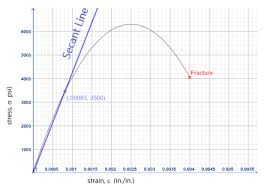## How to Calculate and Solve for Elastic Modulus on Transverse Direction | Composites

The elastic modulus on transverse direction is illustrated by the image below.To compute for elastic modulus on transverse direction, three essential parameters are needed and these parameters are Elastic Modulus of the Fibre (Ef), Elastic Modulus of the Matrix (Em) and Volume Fraction of the Fibre (Vf).

The formula for calculating elastic modulus on transverse direction:

Ec = EmEf/(1 – Vf)Ef + VfEm

Where:

Ec = Elastic Modulus on Transverse Direction
Em = Elastic Modulus of the Fibre
Ef = Elastic Modulus of the Matrix
Vf = Volume Fraction of the Fibre

Given an example;
Find the elastic modulus on transverse direction when the elastic modulus of the fibre is 4, the elastic modulus of the matrix is 8 and the volume fraction of the fibre is 6.

This implies that;

Em = Elastic Modulus of the Fibre = 4
Ef = Elastic Modulus of the Matrix = 8
Vf = Volume Fraction of the Fibre = 6

Ec = EmEf/(1 – Vf)Ef + VfEm
Ec = (4)(8)/(1 – 6)8 + (6)(4)
Ec = (32)/(-5)8 + (24)
Ec = (32)/(-40) + (24)
Ec = (32)/(-16)
Ec = -2

Therefore, the elastic modulus on transverse direction is -2 Pa.